Editor Ratings:
User Ratings:
[Total: 0 Average: 0]

This article covers 5 free online Earth curvature calculator websites. Using these websites, you can calculate the obscured part of a distant object. You can simply input the distance to that object along with the height of the viewpoint and get the results. These calculators also show you the distance to the horizon from the viewpoint.

All these software takes an assumption that Earth is spherical with a radius of 3959 miles and then use the Pythagorean theorem to calculate the distance to the horizon. Then, they use that distance to calculate the Earth curvature. If you don’t know the precise distance to the object, you can use map and navigation services to get that.When you have the distance and Earth curvature, you can use that to calculate the hidden height of a distinct object. So, let’s get started and discuss these calculator websites one by one.

Also read: Find Out How Big Is Solar System In An Interactive Solar System Map

## Walter.bislins.ch

Walter.bislins.ch is a website by a German engineer and educator that offers educational content and tools for free. This website has an Advanced Earth Curvature Calculator which gives detailed results along with other related terms and graphs. The Basics version has 6 following input fields: Observer Height, Target Distance, Target Size, Reflection, Zoom, and View Angle. You can simply enter the Observer Height and Target Distance to calculate the Earth curvature and distance to the horizon along with the obscured height of the target.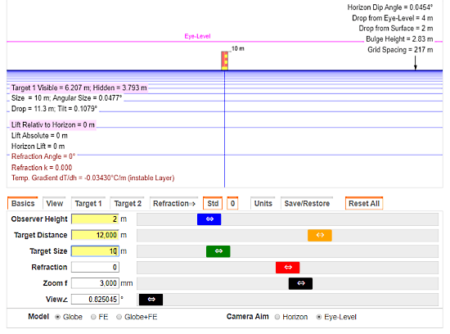If you want to dig deeper, you can adjust the values of all these input fields and get detailed target data and horizon data. This calculator has separate sections for View, Targets, and Refraction where it gives you similar detailed data for those entities. Apart from that, you can change the camera/viewer aim along with globe model and also switch the unit system to Metric or Imperial.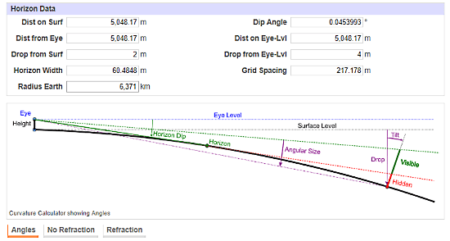Along with the detailed data, this calculator also shows you the graphs of all the angles with and without refraction. It uses color codes to show respective data on the graph which makes it easier to understand.

Try this advanced Earth curvature calculator here.

## MetaBunk.org

MetaBunk.org is an online community where you can find the scientific explanation and (several times) truth behind real-world events, phenomenon, conspiracies and more. This website also offers online tools and educational content on various topics. It has a free online tool where you can calculate the Earth curvature, horizon, bulge, drop, and hidden height.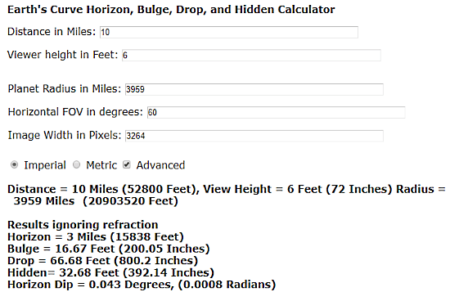This tool has a basic mode where you get two input fields; one to enter the target distance and other to enter the viewer height. When you input both these entities, it gives you the distance between the viewer and the target. The results are accompanied by other additional information showing you the horizon distance, bulge, drop, hidden height and horizon dip. It shows you all this information for both cases; once ignoring the refraction and once with standard refraction value.

Moreover, in the advanced mode, it gives you options to enter the custom values for Earth radius, horizontal FOV, and image width. And, you also get an option to change the unit system as well.

Give this online Earth curvature calculator a try here.

## Horizzn.com

The next Earth curvature calculator website on this list is Horizzn. It is a simple calculator with a neat and elegant design. This calculator has four information fields; Eyesight Level, Distance to Object, Distance to Horizon, and Hidden Object Part (hidden height). Out of these four fields, the first two take user input and the last two provide the output.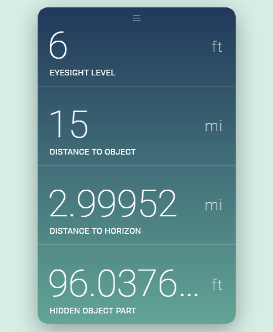Once you enter the Eyesight Level and Distance to Object to this calculator, it immediately gives you the distance to the horizon using the Pythagorean theorem. With that value, it determines the rate at which Earth curves and calculates the hidden height of the object. This calculator supports both, Metric and Imperial unit systems which you can switch by clicking on unit beside any value filed.

You can try this free Earth curvature calculator here.

## OnmiCalculator.com

As the name suggests, OmniCalculator.com is a free website that offers a total of 682 calculators for Math, Physics, Chemistry, Conversion, Finance, Ecology, Health, and more. Under the Physics section, it has an Earth Curvature Calculator which you can use to calculate the obscured part of a distant object.This calculator has three input fields; Distance to the object, Eyesight level, and Distance to the horizon. You can input a value for any two fields to calculate the obscured object height along with the remaining field. It also gives you an option to share the results with others via email, social media, or URL.

Calculate Earth curvature online with OmniCalculator here.

## Dizzib.GitHub.io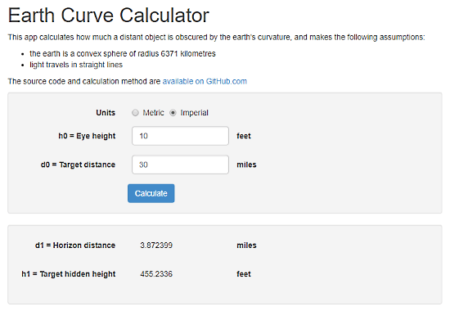Dizzib.GitHub.io is one of the simplest Earth curvature calculator on this list. It has a simple yet comfortable design where you can input your data and get the results. At the top, it has an option to select a unit system for the calculation. Then, there are two input options; one for Eyesight height and other for the Target distance. You can simply enter those two values here and click the Calculate button to get the obscured height of the target along with Horizon distance.

Give this online Earth curvature calculator a try here.

### Closing Words:

These are the 5 free online Earth curvature calculator websites where you can easily calculate the obscured part/height of a distant object. If you are doing a detailed analysis of some kind, then, going with Walter.bislins.ch would be a good idea as it gives you detailed results with analysis. Otherwise, you can go with any other website as per your liking. Go ahead, give them a try and share your thoughts with us in the comments.

 Editor Ratings: User Ratings:[Total: 0 Average: 0] Tags: calculator